# AURIX™ Forum Discussions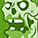Level 1
Level 1

# QSPI BACON calculation of delays

Hi,

I am trying to execute sample program from the vendor for driving external eeprom (25LC640A) using tc234 QSPI0. The below parameters are configured as follows:

BACON.LPRE = 1

fBaud = 200/((49+1)*(1+1+1+1)) = 1 MHz

when I am using the formula as given in user manual TLEAD = TBAUD2 * 4^LPRE * (LEAD+1) I am getting TLEAD as 80 us (10^-6 * 4^4 * 5) but when I measured through oscilloscope I am getting the TLEAD as 2.56 us (approx.). Could you please let me know what I am doing wrong? I believe I am missing something in the calculation.

Many Thanks,
Kumaresh
8 Replies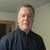Employee
Employee# Re: QSPI BACON calculation of delays

Assuming 200MHz (fBAUD2), I calculate 80 nsec not 80 usec. You should also check the output driver strength for SPI0 pins are set to speed grade 1.Level 1
Level 1

# Re: QSPI BACON calculation of delays

Hi,

I checked the output driver strength and it set to 1. I believe I am confused with the calculation. Could you please elaborate on how you arrived at 80 ns. Thanks for your time.

Regards,
KumareshEmployee
Employee# Re: QSPI BACON calculation of delays

From the User’s Manual

For the calculation of the delay:
TBAUD2 = 1 / fBAUD2

You provided the following parameters
BACON.LPRE = 1
fBAUD2 = 200MHz

Therefore:
TBAUD2 = 1/200MHz = 5e-9 or 5nsec
LPRE = 4^1 = 4
LEAD = 3 + 1 = 4
The value of the LEAD delay is 5e-9 * 4 * 4 = 80e-9 or 80nsecLevel 1
Level 1

# Re: QSPI BACON calculation of delays

Thanks for your detailed reply. I believe I have misunderstanding in substituting the values in BACON.LPRE * BACON.LEAD. As per the manual from my understanding when LPRE = 001b I need to use 4 and TPRE = 3 I need to plug in 4 (pg no 1841 Basic Configuration Register Table). So I plugged in the values as 5e-9 * 4^4 * (4 + 1) and calculated the value as 640 microsec. Could you please comment on how to infer the values from the attached image? In other words my query is what does the value of 0D means in LEAD. Thanks for your time.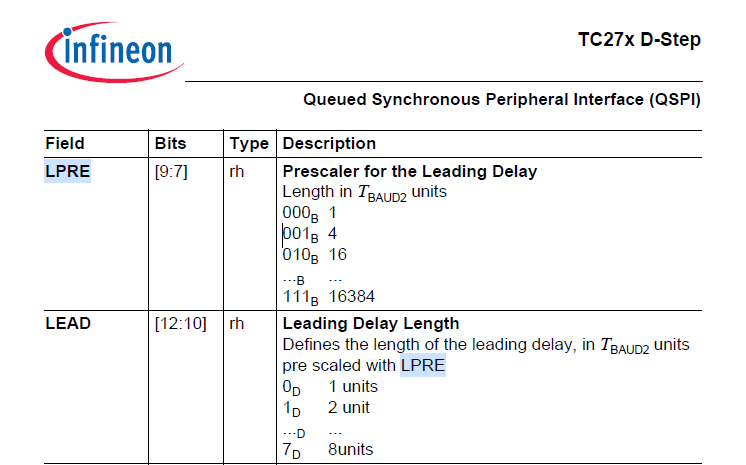Employee
Employee# Re: QSPI BACON calculation of delays

The Formula: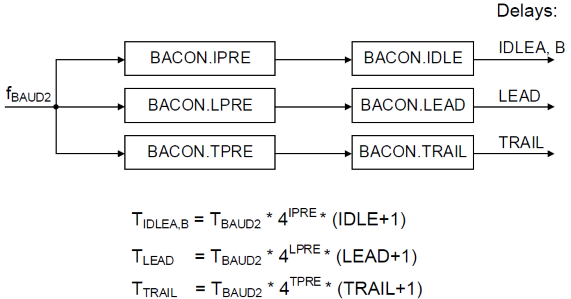BACON.LPRE = 1

Could you please comment on how to infer the values from the attached image? In other words my query is what does the value of 0D means in LEAD

See the calculation of the LEAD delay above.

If the LEAD is 0 decimal, then this what is used in the formula (i.e. LEAD + 1 which is 0 + 1) while the BACON.LEAD = 0 is the register valueLevel 1
Level 1

# Re: QSPI BACON calculation of delays

Thanks Chris for the detailed clarification. It gave me more clearer inference.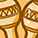Level 3
Level 3

# Re: QSPI BACON calculation of delays

cwunder wrote:
From the User’s Manual

For the calculation of the delay:
TBAUD2 = 1 / fBAUD2

You provided the following parameters
BACON.LPRE = 1
fBAUD2 = 200MHz

Therefore:
TBAUD2 = 1/200MHz = 5e-9 or 5nsec
LPRE = 4^1 = 4
LEAD = 3 + 1 = 4
The value of the LEAD delay is 5e-9 * 4 * 4 = 80e-9 or 80nsec

Hi,

Can you help me to know fBAUD2 is SPI operating frequency or aurix operating frequency.Employee
Employee# Re: QSPI BACON calculation of delays

For the TC2xx devices the fBAUD2 is derived from either the fPLL or fBACK and you can also divide it. It depends on the settings in the CCUCON0 register.